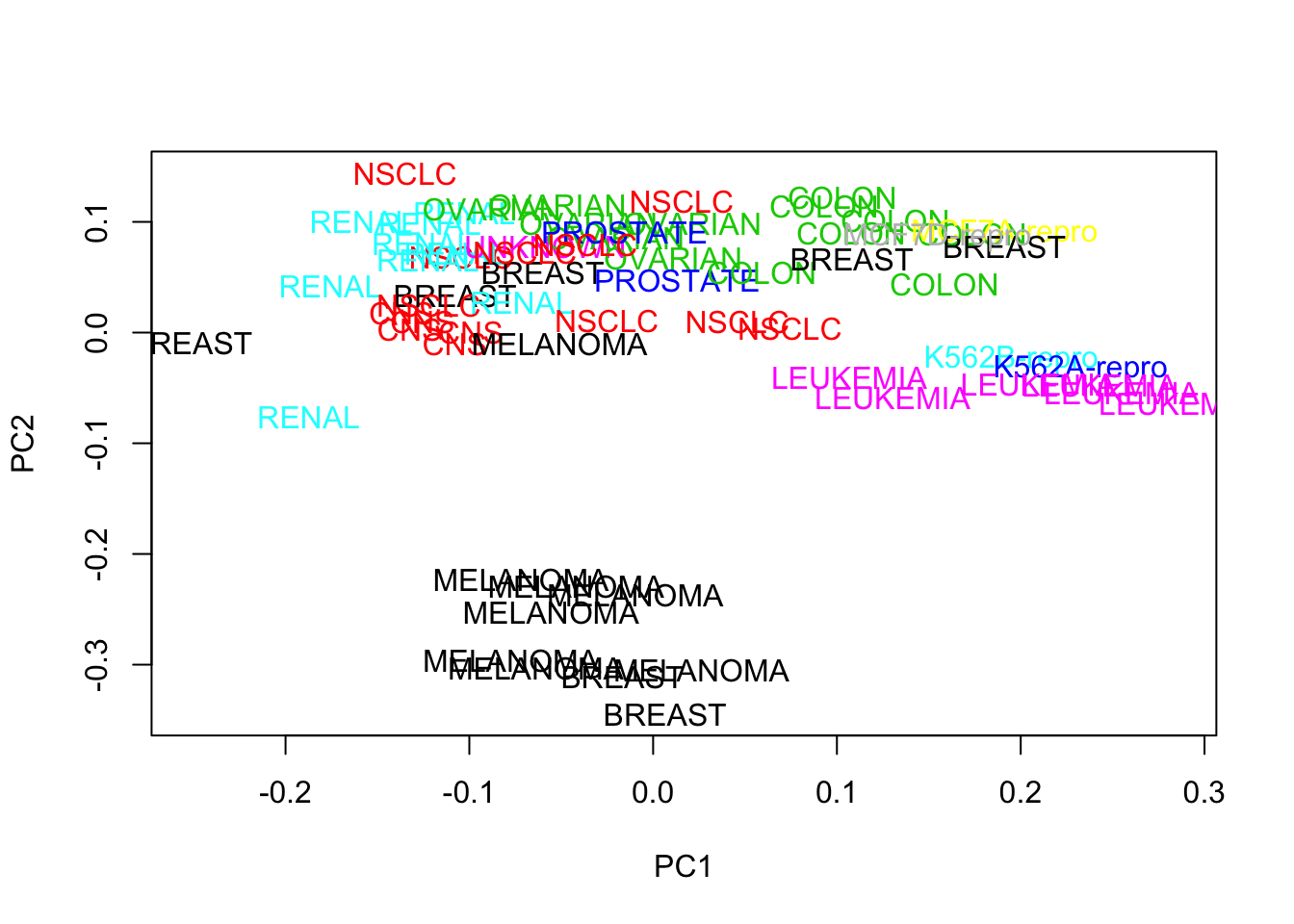### Introduction

Principal component analysis (PCA) and Multidimensional scaling (MDS) are common techniques that are used to visualize high-dimentional data. High-dimentional data are data with features (p) a lot more than observations (n).
That is: `p>>n`

It is very common in genomic data sets where we have tens of thousands of features(genes, DNA methylation regions), but only a handful of samples (genomic are expensive at least for now).

I am going to use a microarray data set to illustrate PCA and MDS, and then show you how to do clustering in R and make pretty heatmaps. It is a pretty old microarray data set, but the skills can be applied to any other high-dimentional genomic data sets. It contains gene expression profile for different cancer types.

Many of the codes are from the R lab of the first big data Summer Institute in the University of Washington. Module 4

If you find anything that I did wrong or explained wrong, please let me know. I am learning also!

``````# install the package if you do not have it.
# install.packages("ISLR")
library(ISLR)

ncidat = t(NCI60\$data)
colnames(ncidat) = NCI60\$labs

dim(ncidat)``````
``##  6830   64``
``unique(colnames(ncidat))``
``````##   "CNS"         "RENAL"       "BREAST"      "NSCLC"       "UNKNOWN"
##   "OVARIAN"     "MELANOMA"    "PROSTATE"    "LEUKEMIA"    "K562B-repro"
##  "K562A-repro" "COLON"       "MCF7A-repro" "MCF7D-repro"``````

First 6 rows, 6 columns of the data:

``ncidat[1:6,1:6]``
``````##      CNS      CNS    CNS         RENAL BREAST    CNS
## 1  0.300 0.679961  0.940  2.800000e-01  0.485  0.310
## 2  1.180 1.289961 -0.040 -3.100000e-01 -0.465 -0.030
## 3  0.550 0.169961 -0.170  6.800000e-01  0.395 -0.100
## 4  1.140 0.379961 -0.040 -8.100000e-01  0.905 -0.460
## 5 -0.265 0.464961 -0.605  6.250000e-01  0.200 -0.205
## 6 -0.070 0.579961  0.000 -1.387779e-17 -0.005 -0.540``````

### PCA - take SVD to get solution.

Singular value decomposition(SVD) is used for PCA.

To get an idea of how svd works, I strongly recommend you to read this and this

We are going to center `(x- mean)` the same gene from different samples, but not scale `(x-mean)/sd` them.
That’s why we transpose the matrix (scale works on columns `?scale`), and then transpose it back. Be aware of whether you should center/scale your data or not. It all depends on the intrinsic properties of your data and the purpose of your analysis. If your data are in the similar range, you do not have to center it.

Usually for a SVD analysis:
X is a n x p matrix (n rows and p columns)
`Xnxp = Unxn %*% Dnxp %*% Vpxp`

One has to be aware that in the microarray data, columns are usually samples(observations n), rows are genes(features p).

we need to first transpose X for the microarray data for the svd analysis

``X = t(scale(t(ncidat),center=TRUE,scale=FALSE))``

### Use svd to get the matrice.

One can use the base R function princomp (default center and scale), but svd gives you more controls.

in a svd analysis, a matrix n x p matrix X is decomposed by `X = U*D*V`:
1.U is an m×n orthogonal matrix.
2.V is an n×n orthogonal matrix.
3.D is an n×n diagonal matrix.

By definition D should be a n x p (64 x 6830) matrix, but in this case, it becomes 64 x 64 matrix.
The reason is that diagonals of D: d1 >= d2 >= d3 >= ….d(r) where r =rank(X), `rank(X) <= min(n,p)`

See the rank of a matrix
The other ds are all zeros. (no variations after d(r)), that’s why D is dropped to 64 x 64 matrix.

We can check it:

``````# we transpose X again for svd
sv = svd(t(X))
U = sv\$u
V = sv\$v
D = sv\$d

## in R calculate the rank of a matrix is by
qr(t(X))\$rank``````
``##  63``
``length(D)``
``##  64``
``min(D)``
``##  1.06447e-13``
``````# the last diagnal in D is very small.
# it is very close to 0, it has to do with the precision of the decimals in computer``````

### let’s plot scatter plot between PCs

PC scatterplots

PCs: Z = XV or Z = UD (U are un-scaled PCs)

Some facts of PCA:

k th column of Z, Z(k), is the k th PC.(the k th pattern)

k th column of V, V(k) is the k th PC loading (feature weights). aka. the k th column of V encodes the associated k th pattern in feature space.

k th column of U, U(k) is the k th PC loading (observation weights). aka. the k th column of U encodes the associated k th pattern in observation space.

Diagnal matrix: D
diagnals in D: d(k) gives the strength of the k th pattern.

Variance explained by k th PC: d(k)^2
Total variance of the data: sum(d(k1)^2 + d(k2)^2 + …..d(k)^2+….)

proportion of variane explained by k th PC: d(k)^2 / sum(d(k1)^2 + d(k2)^2 + …..d(k)^2+….)

Let’s plot U1 vs U2 (plot the loadings). Remember that they are unscaled PCs

``````cols = as.numeric(as.factor(colnames(ncidat)))

plot(U[,1],U[,2],type="n",xlab="PC1",ylab="PC2")
text(U[,1],U[,2],colnames(X),col=cols)``````we see that Melanoma samples are close to each other.

U are un-scaled PCs. We can also plot Z which is scaled PC:
in R `Z<- X%*%V` or `Z<- U %*% diag(D)`

``````par(mfrow=c(1,1))
Z = t(X)%*%V

# plot PC1 vs PC2
plot(Z[,1], Z[,2], type ="n", xlab="PC1", ylab="PC2")
text(Z[,1], Z[,2], colnames(X), col=cols)``````